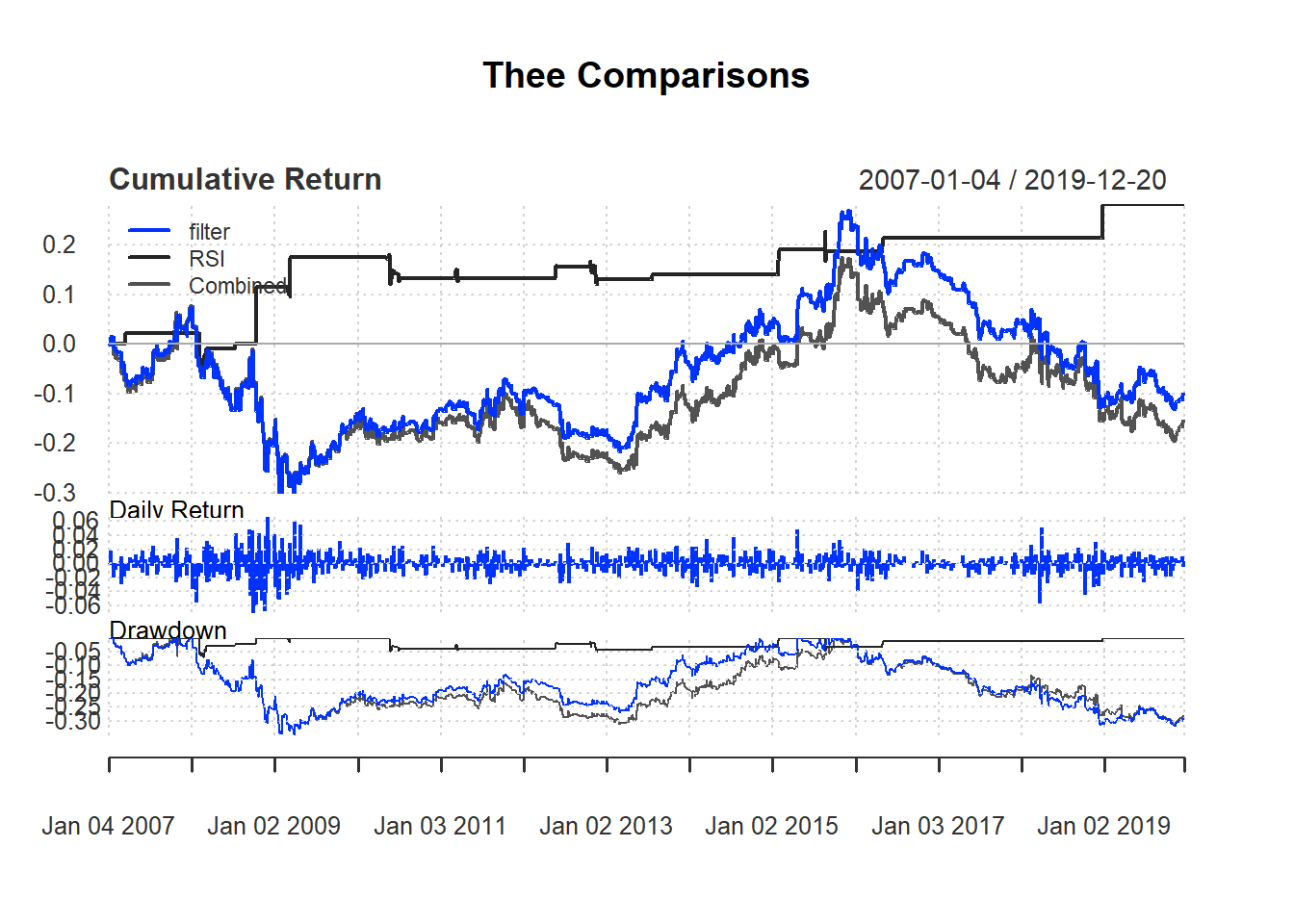## 7.7 Combining two indicators: EMA and RSI

Test the following strategy using EMA and RSI based on day trading:

• Buy signal based on EMA rule.

• Sell signal based on RSI rule.

• We use 14-day RSI and use 70 as threshold for selling.

We will use MSFT to illustrate. We use the closing price and calculate the daily return.

price <- Cl(MSFT)
returnMSFT <- (Cl(MSFT) - Op(MSFT))/Op(MSFT)

Then we calculate the indicator for filter rule and rsi.

delta<-0.005
r <- price/Lag(price) - 1
n <- 14
rsi <- RSI(price, n) 

We calculate the signal based on the trading rule. Note that the trading rule cannot be started until all information is ready. Hence, the first n day have signal value of zero.

signal <-c()
signal[1:n] <-0
for (i in (n+1):length(price)){
if (r[i] > delta){
signal[i]<- 1
} else if (rsi[i] > 70){
signal[i]<- -1
} else
signal[i]<- 0
}
signal<-reclass(signal,price)

EMA.RSI.trade <- Lag(signal)
retall <- cbind(filter.ret,              RSI.ret, EMA.RSI.ret)
charts.PerformanceSummary(retall,
colorset=bluefocus)